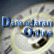See industry averages for fundamentals.

a.     Can you use the same equation to compute growth in operating income?

No. When computing growth in operating income, the equation is slightly different:

Expected growth in operating income = {(Cap ex - Depreciation + Change in non-cash Working capital)/ After-tax Operating Income}* Return on capital

Return on capital = After-tax Operating Income/ (Book value of debt + Book value of equity)

You have to be consistent. When talking about firm value, every input has to be stated in terms of firm value. Thus, in the above equation the retention ratio (which measures reinvestment as a percent of equity income) is replaced by the reinvestment rate (which measures reinvestment as a percent of after-tax operating income) and the return on equity (which is the net income divided by book equity) is replaced by the return on capital (which measures the total return to all investment).

b.     Under what assumptions will this sustainable growth rate also be equal to your expected growth rate?

These equations hold only if the return on equity and capital on existing assets remain unchanged over time. If the return on equity or capital is expected to change over time, there will be a second component to the expected growth rate equation. For instance, assume that your return on capital this year is 10% and that you expect it to improve to 12% next year on exiting assets and that you plan to reinvest 50% of your operating income back next year into new projects on which you expect to make 12%.

Expected growth next year = (.50) (.12) + (.12-.10)/.12 = 26%

You can decompose this growth into 2 parts - growth from new investments (6%) and growth from more efficient use of existing investments (20%). The problem with depending upon the latter is that it is a finite source of growth. At some point in time, your assets will be optimally utilized and you will no longer be able to extract additional growth. That is why you cannot count on the latter in perpetual growth (terminal value).

1. Increasing the amount you reinvest back into the business (reduce the payout ratio or increase the reinvestment rate) will increase the growth rate for any company that is prfitable. Will it also increase value?
No. It depends upon whether the return on capital (equity) is greater that the cost of capital (equity). If the return on capital is less than the cost of capital, increasing the reinvestment rate will increase growth but reduce value. If it is equal, increasing reinvestment will not affect value. It is growth with excess returns that is the source of value.

 1 • 2 • 3 • 4• 5 • 6 • 7 • 8 • 9 • 10 • 11 • 12 • 13 • 14 • 15 • 16 • 17 • 18 • 19 • 20 • 21 • 22 • 23 • 24 • 25 << Previous • Next >>